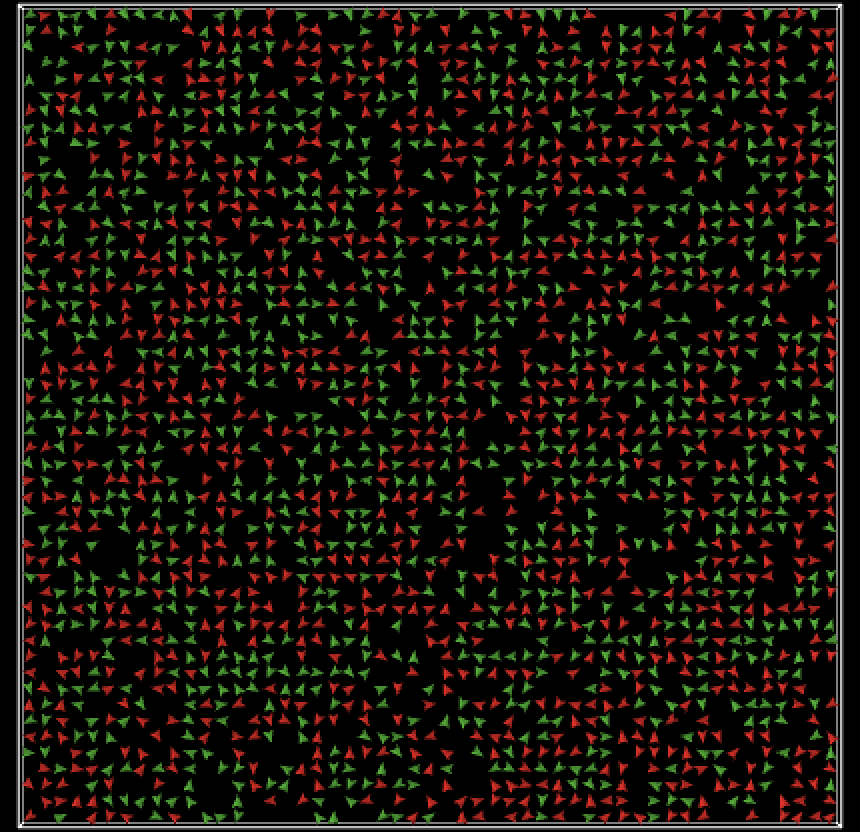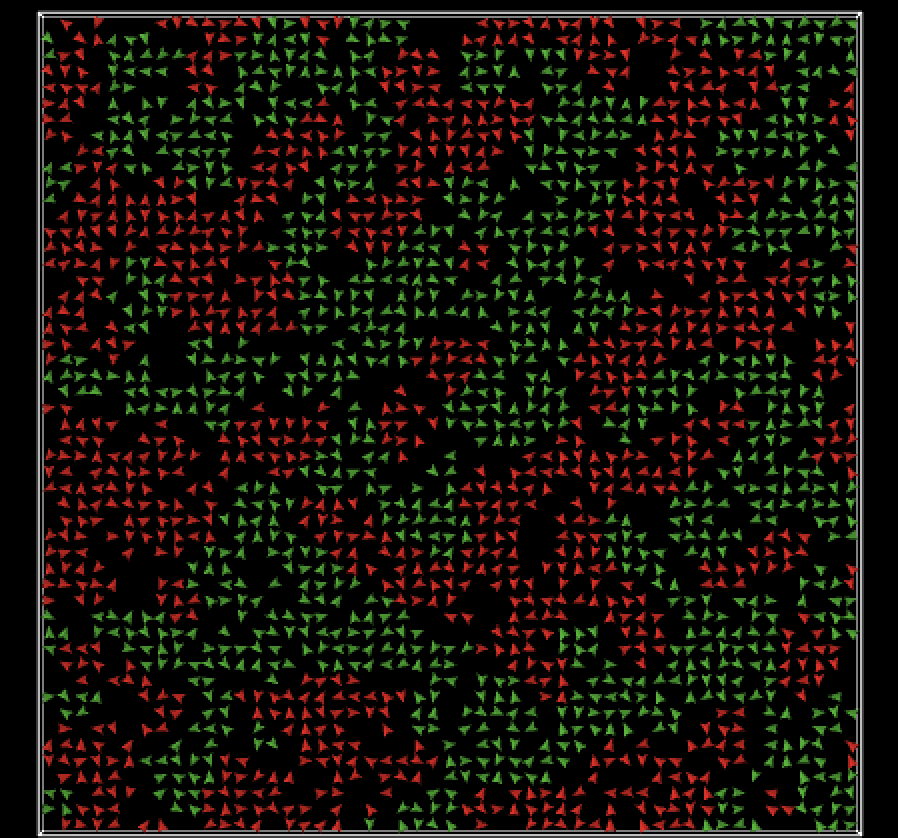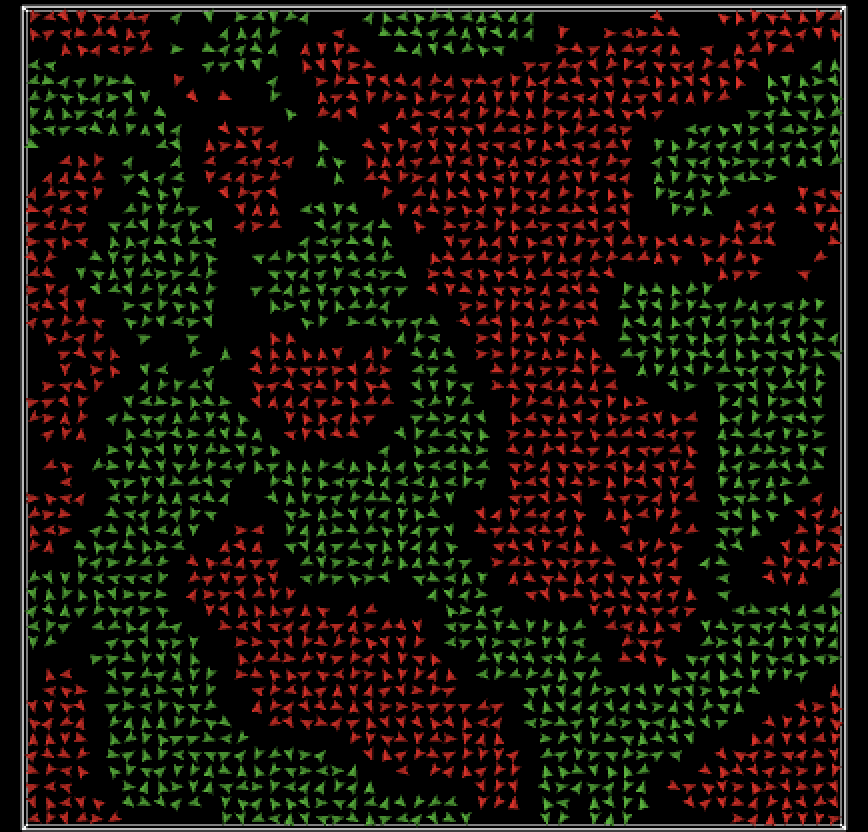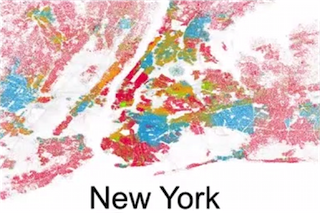2002 字

# 马尔可夫模型下的贫富差距

$$W * p + (1-W) * (1-q)$$

$$W * (1-p) + (1-W) * q$$

1 状态有限（本例中进行二分，富人和穷人） 2 状态可互变（本例中存在一个转移矩阵，表示下一刻状态变换的概率） 3 状态的转移矩阵维持不变（刚才迭代的隐含假设就是状态不变）

$$W * p + (1-W) * (1-q) = W$$

$$\frac{1-W}{W} = \frac{1-p}{1-q}$$3 图片用NetLogo生成

4 纽约隔离图来自网络，如有侵权，烦请告知